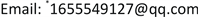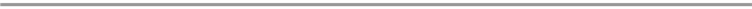﻿ 一类特殊亚纯函数的唯一性象集 Unique Range Sets for a Kind of Special Meromorphic Functions

Pure Mathematics
Vol. 10  No. 02 ( 2020 ), Article ID: 34173 , 7 pages
10.12677/PM.2020.102011

Unique Range Sets for a Kind of Special Meromorphic Functions

Ronghui Li*, Lan Hu

School of Mathematics, Yunnan Normal University, Kunming YunnanReceived: Jan. 21st, 2020; accepted: Feb. 6th, 2020; published: Feb. 13th, 2020ABSTRACT

In this paper, we discuss the problem of unique range sets with fewer elements for a kind of special meromorphic functions. The following result is proved: Let $S=\left\{z:{z}^{6}+{z}^{5}+1=0\right\}$ be a set such that two nonconstant meromorphic functions $f\left(z\right)$ and $g\left(z\right)$ satisfy ${E}_{f}\left(S\right)={E}_{g}\left(S\right)$. If we attach certain conditions to $f\left(z\right)$ and $g\left(z\right)$, then $f\left(z\right)\equiv g\left(z\right)$.

Keywords:Meromorphic Functions, Shared Set, Uniqueness1. 引言及主要结果

$f\left(z\right)$ 为开平面 $\overline{)C}$ 上的非常数亚纯函数， $a\left(z\right)$ (可恒为 $\infty$ )是一个亚纯函数。当 $a\left(z\right)\overline{)\equiv }\infty$ 时，若，则称 $a\left(z\right)$$f\left(z\right)$ 的小函数。当时，也称 $a\left(z\right)$$f\left(z\right)$ 的小函数。

$f\left(z\right)$ 为开平面 $\overline{)C}$ 上的非常数亚纯函数，S是扩充复平面 $\stackrel{^}{\overline{)C}}$ 上的一个非空集合且具有不同的元素。令 

${E}_{f}\left(S\right)=\underset{a\in S}{\cup }\left\{z|f\left(z\right)=a\right\}$ (这里m重a值点记m次)。

$f\left(z\right)$$g\left(z\right)$ 为开平面 $\overline{)C}$ 上的两个非常数亚纯函数，如果 ${E}_{f}\left(S\right)={E}_{g}\left(S\right)$，则称 $f\left(z\right)$$g\left(z\right)$ 以S为CM分担值集；如果对于 $\forall {z}^{*}\in \left\{z|f\left(z\right)=a\right\}$ 都有 ${z}^{*}$ 作为方程 $f\left(z\right)=a$ 的根的重数等于 ${z}^{*}$ 作为方程 $g\left(z\right)=a$ 的根的重数，则称 $f\left(z\right)$$g\left(z\right)$ 以a为CM分担值，其中 $a\in \overline{)C}$

F. Gross和杨重骏在文献  中证明了定理：

1976年，F. Gross在文献  中提出这样一个问题：

1993年，仪洪勋在文献  中构造出了含有15个元素的整函数的CM型唯一性象集，给F. Gross提出的问题一个肯定的回答，并于1995年在文  中建立了含有7个元素的整函数CM型唯一性象集。并在文献  中构造了一个含有11个点的亚纯函数唯一性象集。

2000年，杨力在文  中得到了一个含有5个元素的集合为有穷非正整数下级整函数的唯一性象集。

2001年，王新利推广了定理C并得到了：

2003年，徐炎在文  中改进了定理C得到了：

2004年，段曦盛在文  中得到了含有10个点的亚纯函数精简唯一性象集。2011年，白小甜在文  中也得到了含有10个点的亚纯函数精简唯一性象集。

$\stackrel{¯}{N}\left(r,f\right)+\stackrel{¯}{N}\left(r,g\right)\le \lambda \left(T\left(r,f\right)+T\left(r,g\right)\right)+{S}^{*}\left(r,f\right)+{S}^{*}\left(r,g\right)$ (其中 $\lambda <\frac{1}{4}$ )，

$\stackrel{¯}{N}\left(r,\frac{1}{f}\right)\le m\left(r,\frac{1}{f}\right)+{S}^{*}\left(r,f\right)$,

$\stackrel{¯}{N}\left(r,f\right)+\stackrel{¯}{N}\left(r,g\right)\le \lambda \left(T\left(r,f\right)+T\left(r,g\right)\right)+{S}^{*}\left(r,f\right)+{S}^{*}\left(r,g\right)$ (其中 $\lambda <\frac{1}{4}$ )，

$\stackrel{¯}{N}\left(r,\frac{1}{f}\right)\le m\left(r,\frac{1}{f}\right)+{S}^{*}\left(r,f\right)$,$\stackrel{¯}{N}\left(r,\frac{1}{g}\right)\le m\left(r,\frac{1}{g}\right)+{S}^{*}\left(r,g\right)$,

2. 几个引理

$T\left(r,Q\left(f\right)\right)=pT\left(r,f\right)+{S}^{*}\left(r,f\right)$.

${N}_{2}\left(r,\frac{1}{f}\right)+{N}_{2}\left(r,\frac{1}{g}\right)+{N}_{2}\left(r,f\right)+{N}_{2}\left(r,g\right)<\left(\mu +ο\left(1\right)\right)T\left(r\right)\left(r\in I\right)$,

$T\left(r,R\left(f\right)\right)=\mathrm{max}\left\{p,q\right\}T\left(r,f\right)+{S}^{*}\left(r,f\right)$.

3. 定理1的证明

$F\left(z\right)=-{f}^{5}\left(z\right)\left(f\left(z\right)+1\right)$,$G\left(z\right)=-{g}^{5}\left(z\right)\left(g\left(z\right)+1\right)$. (1)

$F\left(z\right)$$G\left(z\right)$ 也为开平面 $\overline{)C}$ 上的非常数亚纯函数，且以1为CM分担值。于是由引理1、定理条件及(1)式可得：

$T\left(r,F\right)=6T\left(r,f\right)+{S}^{*}\left(r,f\right)$, (2)

$T\left(r,G\right)=6T\left(r,g\right)+{S}^{*}\left(r,g\right)$, (3)

$\begin{array}{c}{N}_{2}\left(r,\frac{1}{F}\right)\le 2\stackrel{¯}{N}\left(r,\frac{1}{f}\right)+N\left(r,\frac{1}{f+1}\right)\\ \le \stackrel{¯}{N}\left(r,\frac{1}{f}\right)+m\left(r,\frac{1}{f}\right)+N\left(r,\frac{1}{f+1}\right)+{S}^{*}\left(r,f\right)\\ \le 2T\left(r,f\right)+Ο\left(1\right)\end{array}$, (4)

${N}_{2}\left(r,F\right)=2\stackrel{¯}{N}\left(r,f\right)$, (5)

${N}_{2}\left(r,\frac{1}{G}\right)\le 2\stackrel{¯}{N}\left(r,\frac{1}{g}\right)+N\left(r,\frac{1}{g+1}\right)\le 3T\left(r,g\right)+Ο\left(1\right)$, (6)

${N}_{2}\left(r,G\right)=2\stackrel{¯}{N}\left(r,g\right)$. (7)

$\begin{array}{l}{N}_{2}\left(r,\frac{1}{F}\right)+{N}_{2}\left(r,\frac{1}{G}\right)+{N}_{2}\left(r,F\right)+{N}_{2}\left(r,G\right)\\ \le 2T\left(r,f\right)+3T\left(r,g\right)+2\lambda \left(T\left(r,f\right)+T\left(r,g\right)\right)+{S}^{*}\left(r,f\right)+{S}^{*}\left(r,g\right)\\ \le \left(2+2\lambda \right)T\left(r,f\right)+\left(3+2\lambda \right)T\left(r,g\right)+{S}^{*}\left(r,f\right)+{S}^{*}\left(r,g\right)\\ =\frac{\left(2+2\lambda \right)}{6}T\left(r,F\right)+\frac{\left(3+2\lambda \right)}{6}T\left(r,G\right)+{S}^{*}\left(r,F\right)+{S}^{*}\left(r,G\right)\\ \le \left(\frac{5+4\lambda }{6}+ο\left(1\right)\right)T\left(r\right)\left(r\notin E\right)\end{array}$, (8)

$F\cdot G\equiv 1$，则有

${f}^{5}{g}^{5}\left(f+1\right)\left(g+1\right)\equiv 1$. (9)

$5p=6q$, (10)

$\stackrel{¯}{N}\left(r,\frac{1}{f}\right)\le \frac{1}{p}N\left(r,\frac{1}{f}\right)\le \frac{1}{6}N\left(r,\frac{1}{f}\right)\le \frac{1}{6}T\left(r,f\right)+Ο\left(1\right)$, (11)

${z}_{1}$$f+1$ 的零点，则由(9)式知 ${z}_{1}$ 至少为 $f+1$ 的6重零点，从而有

$\stackrel{¯}{N}\left(r,\frac{1}{f+1}\right)\le \frac{1}{6}N\left(r,\frac{1}{f+1}\right)\le \frac{1}{6}T\left(r,f\right)+Ο\left(1\right)$, (12)

$\stackrel{¯}{N}\left(r,\frac{1}{g}\right)\le \frac{1}{p}N\left(r,\frac{1}{g}\right)\le \frac{1}{6}N\left(r,\frac{1}{g}\right)\le \frac{1}{6}T\left(r,g\right)+Ο\left(1\right)$, (13)

$\stackrel{¯}{N}\left(r,\frac{1}{g+1}\right)\le \frac{1}{6}N\left(r,\frac{1}{g+1}\right)\le \frac{1}{6}T\left(r,g\right)+Ο\left(1\right)$,(14)

$\begin{array}{c}T\left(r,f\right)+T\left(r,g\right)<\stackrel{¯}{N}\left(r,f\right)+\stackrel{¯}{N}\left(r,g\right)+\stackrel{¯}{N}\left(r,\frac{1}{f}\right)+\stackrel{¯}{N}\left(r,\frac{1}{f+1}\right)\\ \text{\hspace{0.17em}}\text{\hspace{0.17em}}+\stackrel{¯}{N}\left(r,\frac{1}{g}\right)+\stackrel{¯}{N}\left(r,\frac{1}{g+1}\right)+{S}^{*}\left(r,f\right)+{S}^{*}\left(r,g\right)\\ \le \lambda \left(T\left(r,f\right)+T\left(r,g\right)\right)+\frac{1}{3}\left(T\left(r,f\right)+T\left(r,g\right)\right)+{S}^{*}\left(r,f\right)+{S}^{*}\left(r,g\right)\\ =\left(\lambda +\frac{1}{3}\right)\left(T\left(r,f\right)+T\left(r,g\right)\right)+{S}^{*}\left(r,f\right)+{S}^{*}\left(r,g\right)\\ <\frac{7}{12}\left(T\left(r,f\right)+T\left(r,g\right)\right)+{S}^{*}\left(r,f\right)+{S}^{*}\left(r,g\right)\end{array}$,

$F\equiv G$，则有

${f}^{6}+{f}^{5}={g}^{6}+{g}^{5}$, (15)

$h=\frac{f}{g}$，则(15)式可变形为

$\left({h}^{6}-1\right)g+\left({h}^{5}-1\right)\equiv 0$, (16)

$g\equiv -\frac{{h}^{5}-1}{{h}^{6}-1}=-\frac{\left(h-u\right)\left(h-{u}^{2}\right)\left(h-{u}^{3}\right)\left(h-{u}^{4}\right)}{\left(h-v\right)\left(h-{v}^{2}\right)\left(h-{v}^{3}\right)\left(h-{v}^{4}\right)\left(h-{v}^{5}\right)}$, (17)

$f\equiv -\frac{\frac{1}{{h}^{5}}-1}{\frac{1}{{h}^{6}}-1}=-\frac{\left(\frac{1}{h}-u\right)\left(\frac{1}{h}-{u}^{2}\right)\left(\frac{1}{h}-{u}^{3}\right)\left(\frac{1}{h}-{u}^{4}\right)}{\left(\frac{1}{h}-v\right)\left(\frac{1}{h}-{v}^{2}\right)\left(\frac{1}{h}-{v}^{3}\right)\left(\frac{1}{h}-{v}^{4}\right)\left(\frac{1}{h}-{v}^{5}\right)}$, (18)

$T\left(r,g\right)=5T\left(r,h\right)+{S}^{*}\left(r,h\right)$, (19)

$\stackrel{¯}{N}\left(r,g\right)=\underset{j=1}{\overset{5}{\sum }}\stackrel{¯}{N}\left(r,\frac{1}{h-{v}^{j}}\right)$, (20)

$T\left(r,f\right)=5T\left(r,\frac{1}{h}\right)+{S}^{*}\left(r,h\right)=5T\left(r,h\right)+{S}^{*}\left(r,h\right)$,(21)

$\stackrel{¯}{N}\left(r,f\right)=\underset{j=1}{\overset{5}{\sum }}\stackrel{¯}{N}\left(r,\frac{1}{\frac{1}{h}-{v}^{j}}\right)$, (22)

$3T\left(r,h\right)<\underset{j=1}{\overset{5}{\sum }}\stackrel{¯}{N}\left(r,\frac{1}{h-{v}^{j}}\right)+{S}^{*}\left(r,h\right)=\stackrel{¯}{N}\left(r,g\right)+{S}^{*}\left(r,h\right)$, (23)

$3T\left(r,\frac{1}{h}\right)<\underset{j=1}{\overset{5}{\sum }}\stackrel{¯}{N}\left(r,\frac{1}{\frac{1}{h}-{v}^{j}}\right)+{S}^{*}\left(r,h\right)=\stackrel{¯}{N}\left(r,f\right)+{S}^{*}\left(r,h\right)$, (24)

$T\left(r,\frac{1}{h}\right)=T\left(r,h\right)+Ο\left(1\right)$,(25)

$\begin{array}{c}6T\left(r,h\right)<\stackrel{¯}{N}\left(r,f\right)+\stackrel{¯}{N}\left(r,g\right)+{S}^{*}\left(r,h\right)\\ \le \lambda \left(T\left(r,f\right)+T\left(r,g\right)\right)+{S}^{\ast }\left(r,h\right)\\ <10\lambda T\left(r,h\right)+{S}^{\ast }\left(r,h\right)<\frac{5}{2}T\left(r,h\right)+{S}^{\ast }\left(r,h\right)\end{array}$,

Unique Range Sets for a Kind of Special Meromorphic Functions[J]. 理论数学, 2020, 10(02): 65-71. https://doi.org/10.12677/PM.2020.102011

1. 1. 杨乐. 值分布论及其新研究[M]. 北京: 科学出版社, 1982.

2. 2. 仪洪勋, 杨重骏. 亚纯函数唯一性理论[M]. 北京: 科学出版社, 1995.

3. 3. Gross, F. and Yang, C.C. (1982) On Preimage and Range Sets of Meromorphic Functions. Proceedings of the Japan Academy, Ser. A, Mathematical Sciences, 58, 17-20.https://doi.org/10.3792/pjaa.58.17

4. 4. Gross, F. (1977) Factorization of Meromorphic Function and Some Open Problems. In: Complex Analysis, Lecture Notes in Math, Vol. 599, Springer, Berlin, Heidelberg, New York, 51-69.https://doi.org/10.1007/BFb0096825

5. 5. Yi, H.X. (1995) On a Question of Gross. Science in China (Ser A), 38, 8-16.

6. 6. Yi, H.X. (1995) A Question of Gross and the Uniqueness of Entire Functions. Nagoya Mathematical Journal, 138, 169-177.https://doi.org/10.1017/S0027763000005225

7. 7. 仪洪勋. 关于亚纯函数的精简唯一性象集[J]. 山东大学学报(自然科学版), 1998, 33(4): 361-369.

8. 8. Fang, M.L. and Hua, X.H. (1998) Meromorpic Functions That Share One Finite Set CM. Journal of Nanjing University Mathematical Biquarterly, 15, 16-22.

9. 9. 杨力. 一类整函数的唯一性象集[J]. 纺织高校基础科学学报, 2000, 13(3): 198-201.

10. 10. 王新利. 具有一个CM公共值集的亚纯函数[J]. 山东大学学报, 2001, 36(1): 5-10.

11. 11. Xu, Y. (2003) Meromorphic Functions Sharing One Finite Set. Computers and Mathematics with Applications, 45, 1489-1495.https://doi.org/10.1016/S0898-1221(03)00132-9

12. 12. 段曦盛. 亚纯函数的几个唯一性定[D]: [硕士学位论文]. 重庆: 重庆大学, 2004.

13. 13. 白小甜. 关于亚纯函数分担公共值集的一些结果[D]: [博士学位论文]. 济南: 山东大学, 2011.

14. 14. 熊坚. 关于亚纯函数的分担值集与唯一性[D]: [硕士学位论文]. 昆明: 云南师范大学, 2008.

15. 15. Yi, H.X. (1994) Unicity Theorems for Entire Functions. Kodai Mathematical Journal, 17, 133-141.https://doi.org/10.2996/kmj/1138039903

NOTES

*通讯作者。